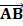# The moment about the pointof the force represented in magnitude and positionwhere the pointsandhave the coordinates (1, 2,andrespectively, is a)b)c)d)## Question ID - 53338 :- The moment about the pointof the force represented in magnitude and positionwhere the pointsandhave the coordinates (1, 2,andrespectively, is a)b)c)d)3537

(a)

ForceMoment of forcewith respect toNow,Next Question :
 A thin rod of lengthis lying along the-axis with its ends atand. Its linear density (mass\length) varies withas k, whencan be zero or any positive number. If the positionof the centre of mass of the rod is plotted against, which of the following  graphs best approximates the dependence ofon? a)b)c)d)